# Pedestrian and Bicyclist Classification Using Deep Learning

This example shows how to classify pedestrians and bicyclists based on their micro-Doppler characteristics using a deep learning network and time-frequency analysis.

The movements of different parts of an object placed in front of a radar produce micro-Doppler signatures that can be used to identify the object. This example uses a convolutional neural network (CNN) to identify pedestrians and bicyclists based on their signatures.

This example trains the deep learning network using simulated data and then examines how the network performs at classifying two cases of overlapping signatures.

### Synthetic Data Generation by Simulation

The data used to train the network is generated using `backscatterPedestrian` and `backscatterBicyclist` from Radar Toolbox™. These functions simulate the radar backscattering of signals reflected from pedestrians and bicyclists, respectively.

The helper function `helperBackScatterSignals` generates a specified number of pedestrian, bicyclist, and car radar returns. Because the purpose of the example is to classify pedestrians and bicyclists, this example considers car signatures as noise sources only. To get an idea of the classification problem to solve, examine one realization of a micro-Doppler signature from a pedestrian, a bicyclist, and a car. (For each realization, the return signals have dimensions ${\mathit{N}}_{\mathrm{fast}}$-by-${\mathit{N}}_{\mathrm{slow}}$, where ${\mathit{N}}_{\mathrm{fast}}$ is the number of fast-time samples and ${\mathit{N}}_{\mathrm{slow}}$ is the number of slow-time samples. See Radar Data Cube for more information.)

```numPed = 1; % Number of pedestrian realizations numBic = 1; % Number of bicyclist realizations numCar = 1; % Number of car realizations [xPedRec,xBicRec,xCarRec,Tsamp] = helperBackScatterSignals(numPed,numBic,numCar);```

The helper function `helperDopplerSignatures` computes the short-time Fourier transform (STFT) of a radar return to generate the micro-Doppler signature. To obtain the micro-Doppler signatures, use the helper functions to apply the STFT and a preprocessing method to each signal.

```[SPed,T,F] = helperDopplerSignatures(xPedRec,Tsamp); [SBic,~,~] = helperDopplerSignatures(xBicRec,Tsamp); [SCar,~,~] = helperDopplerSignatures(xCarRec,Tsamp);```

Plot the time-frequency maps for the pedestrian, bicyclist, and car realizations.

```% Plot the first realization of objects figure subplot(1,3,1) imagesc(T,F,SPed(:,:,1)) ylabel('Frequency (Hz)') title('Pedestrian') axis square xy subplot(1,3,2) imagesc(T,F,SBic(:,:,1)) xlabel('Time (s)') title('Bicyclist') axis square xy subplot(1,3,3) imagesc(T,F,SCar(:,:,1)) title('Car') axis square xy```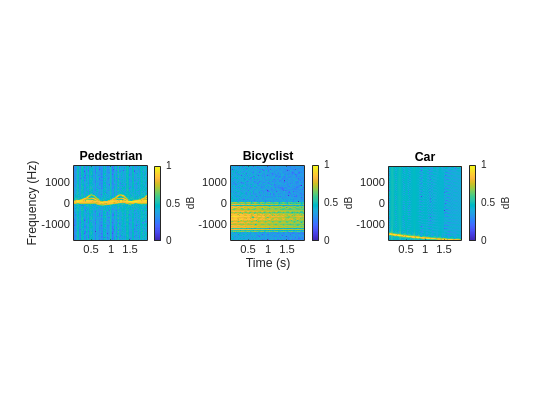The normalized spectrograms (STFT absolute values) show that the three objects have quite distinct signatures. Specifically, the spectrograms of the pedestrian and the bicyclist have rich micro-Doppler signatures caused by the swing of arms and legs and the rotation of wheels, respectively. By contrast, in this example, the car is modeled as a point target with rigid body, so the spectrogram of the car shows that the short-term Doppler frequency shift varies little, indicating little micro-Doppler effect.

#### Combining Objects

Classifying a single realization as a pedestrian or bicyclist is relatively simple because the pedestrian and bicyclist micro-Doppler signatures are dissimilar. However, classifying multiple overlapping pedestrians or bicyclists, with the addition of Gaussian noise or car noise, is much more difficult.

If multiple objects exist in the detection region of the radar at the same time, the received radar signal is a summation of the detection signals from all the objects. As an example, generate the received radar signal for a pedestrian and bicyclist with Gaussian background noise.

```% Configure Gaussian noise level at the receiver rx = phased.ReceiverPreamp('Gain',25,'NoiseFigure',10); xRadarRec = complex(zeros(size(xPedRec))); for ii = 1:size(xPedRec,3) xRadarRec(:,:,ii) = rx(xPedRec(:,:,ii) + xBicRec(:,:,ii)); end```

Then obtain micro-Doppler signatures of the received signal by using the STFT.

```[S,~,~] = helperDopplerSignatures(xRadarRec,Tsamp); figure imagesc(T,F,S(:,:,1)) % Plot the first realization axis xy xlabel('Time (s)') ylabel('Frequency (Hz)') title('Spectrogram of a Pedestrian and a Bicyclist')```Because the pedestrian and bicyclist signatures overlap in time and frequency, differentiating between the two objects is difficult.

### Generate Training Data

In this example, you train a CNN by using data consisting of simulated realizations of objects with varying properties—for example, bicyclists pedaling at different speeds and pedestrians with different heights walking at different speeds. Assuming the radar is fixed at the origin, in one realization, one object or multiple objects are uniformly distributed in a rectangular area of [5, 45] and [–10, 10] meters along the X and Y axes, respectively.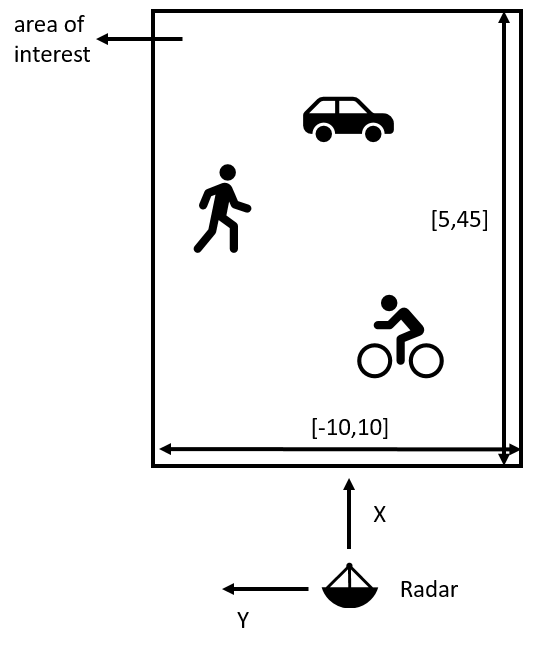The other properties of the three objects that are randomly tuned are as follows:

1) Pedestrians

• Height — Uniformly distributed in the interval of [1.5, 2] meters

• Heading — Uniformly distributed in the interval of [–180, 180] degrees

• Speed — Uniformly distributed in the interval of [0, 1.4h] meters/second, where `h` is the height value

2) Bicyclists

• Heading — Uniformly distributed in the interval of [–180, 180] degrees

• Speed — Uniformly distributed in the interval of [1, 10] meters/second

• Gear transmission ratio — Uniformly distributed in the interval of [0.5, 6]

• Pedaling or coasting — 50% probability of pedaling (coasting means that the cyclist is moving without pedaling)

3) Cars

• Velocity — Uniformly distributed in the interval of [0, 10] meters/second along the X and Y directions

The input to the convolutional network is micro-Doppler signatures consisting of spectrograms expressed in decibels and normalized to [0, 1], as shown in this figure: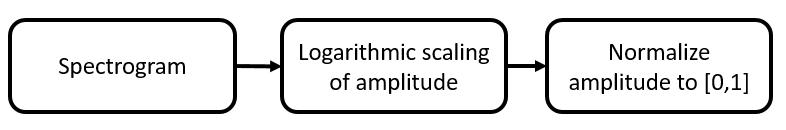Radar returns originate from different objects and different parts of objects. Depending on the configuration, some returns are much stronger than others. Stronger returns tend to obscure weaker ones. Logarithmic scaling augments the features by making return strengths comparable. Amplitude normalization helps the CNN converge faster.

The data set contains realizations of the following scenes:

• One pedestrian present in the scene

• One bicyclist present in the scene

• One pedestrian and one bicyclist present in the scene

• Two pedestrians present in the scene

• Two bicyclists present in the scene

The data for this example consists of 20,000 pedestrian, 20,000 bicyclist, and 12,500 car signals generated by using the helper functions `helperBackScatterSignals` and `helperDopplerSignatures`. The signals are divided into two data sets: one without car noise samples and one with car noise samples.

For the first data set (without car noise), the pedestrian and bicyclist signals were combined, Gaussian noise was added, and micro-Doppler signatures were computed to generate 5000 signatures for each of the five scenes to be classified.

In each category, 80% of the signatures (that is, 4000 signatures) are reserved for the training data set while 20% of the signatures (that is, 1000 signatures) are reserved for the test data set.

To generate the second data set (with car noise), the procedure for the first data set was followed, except that car noise was added to 50% of the signatures. The proportion of signatures with and without car noise is the same in the training and test data sets.

Download and unzip the data in your temporary directory, whose location is specified by MATLAB®'s `tempdir` command. The data has a size of 21 GB and the download process may take some time. If you have the data in a folder different from `tempdir`, change the directory name in the subsequent instructions.

```% Download the data dataURL = 'https://ssd.mathworks.com/supportfiles/SPT/data/PedBicCarData.zip'; saveFolder = fullfile(tempdir,'PedBicCarData'); zipFile = fullfile(tempdir,'PedBicCarData.zip'); if ~exist(zipFile,'file') websave(zipFile,dataURL); elseif ~exist(saveFolder,'dir') % Unzip the data unzip(zipFile,tempdir) end```

The data files are as follows:

• `trainDataNoCar.mat` contains the training data set `trainDataNoCar` and its label set `trainLabelNoCar`.

• `testDataNoCar.mat` contains the test data set `testDataNoCar` and its label set `testLabelNoCar`.

• `trainDataCarNoise.mat` contains the training data set `trainDataCarNoise` and its label set `trainLabelCarNoise`.

• `testDataCarNoise.mat` contains the test data set `testDataCarNoise` and its label set `testLabelCarNoise`.

• `TF.mat` contains the time and frequency information for the micro-Doppler signatures.

### Network Architecture

Create a CNN with five convolution layers and one fully connected layer. The first four convolution layers are followed by a batch normalization layer, a rectified linear unit (ReLU) activation layer, and a max pooling layer. In the last convolution layer, the max pooling layer is replaced by an average pooling layer. The output layer is a classification layer after softmax activation. For network design guidance, see Deep Learning Tips and Tricks (Deep Learning Toolbox).

```layers = [ imageInputLayer([size(S,1),size(S,2),1],'Normalization','none') convolution2dLayer(10,16,'Padding','same') batchNormalizationLayer reluLayer maxPooling2dLayer(10,'Stride',2) convolution2dLayer(5,32,'Padding','same') batchNormalizationLayer reluLayer maxPooling2dLayer(10,'Stride',2) convolution2dLayer(5,32,'Padding','same') batchNormalizationLayer reluLayer maxPooling2dLayer(10,'Stride',2) convolution2dLayer(5,32,'Padding','same') batchNormalizationLayer reluLayer maxPooling2dLayer(5,'Stride',2) convolution2dLayer(5,32,'Padding','same') batchNormalizationLayer reluLayer averagePooling2dLayer(2,'Stride',2) fullyConnectedLayer(5) softmaxLayer classificationLayer]```
```layers = 24x1 Layer array with layers: 1 '' Image Input 400x144x1 images 2 '' Convolution 16 10x10 convolutions with stride [1 1] and padding 'same' 3 '' Batch Normalization Batch normalization 4 '' ReLU ReLU 5 '' Max Pooling 10x10 max pooling with stride [2 2] and padding [0 0 0 0] 6 '' Convolution 32 5x5 convolutions with stride [1 1] and padding 'same' 7 '' Batch Normalization Batch normalization 8 '' ReLU ReLU 9 '' Max Pooling 10x10 max pooling with stride [2 2] and padding [0 0 0 0] 10 '' Convolution 32 5x5 convolutions with stride [1 1] and padding 'same' 11 '' Batch Normalization Batch normalization 12 '' ReLU ReLU 13 '' Max Pooling 10x10 max pooling with stride [2 2] and padding [0 0 0 0] 14 '' Convolution 32 5x5 convolutions with stride [1 1] and padding 'same' 15 '' Batch Normalization Batch normalization 16 '' ReLU ReLU 17 '' Max Pooling 5x5 max pooling with stride [2 2] and padding [0 0 0 0] 18 '' Convolution 32 5x5 convolutions with stride [1 1] and padding 'same' 19 '' Batch Normalization Batch normalization 20 '' ReLU ReLU 21 '' Average Pooling 2x2 average pooling with stride [2 2] and padding [0 0 0 0] 22 '' Fully Connected 5 fully connected layer 23 '' Softmax softmax 24 '' Classification Output crossentropyex ```

Specify the optimization solver and the hyperparameters to train the CNN using `trainingOptions`. This example uses the ADAM optimizer and a mini-batch size of 128. Train the network using either a CPU or GPU. Using a GPU requires Parallel Computing Toolbox™. To see which GPUs are supported, see GPU Support by Release (Parallel Computing Toolbox). For information on other parameters, see `trainingOptions` (Deep Learning Toolbox). This example uses a GPU for training.

```options = trainingOptions('adam', ... 'ExecutionEnvironment','gpu',... 'MiniBatchSize',128, ... 'MaxEpochs',30, ... 'InitialLearnRate',1e-2, ... 'LearnRateSchedule','piecewise', ... 'LearnRateDropFactor',0.1, ... 'LearnRateDropPeriod',10, ... 'Shuffle','every-epoch', ... 'Verbose',false, ... 'Plots','training-progress');```

### Classify Signatures Without Car Noise

Load the data set without car noise and use the helper function `helperPlotTrainData` to plot one example of each of the five categories in the training data set,

```load(fullfile(tempdir,'PedBicCarData','trainDataNoCar.mat')) % load training data set load(fullfile(tempdir,'PedBicCarData','testDataNoCar.mat')) % load test data set load(fullfile(tempdir,'PedBicCarData','TF.mat')) % load time and frequency information helperPlotTrainData(trainDataNoCar,trainLabelNoCar,T,F)```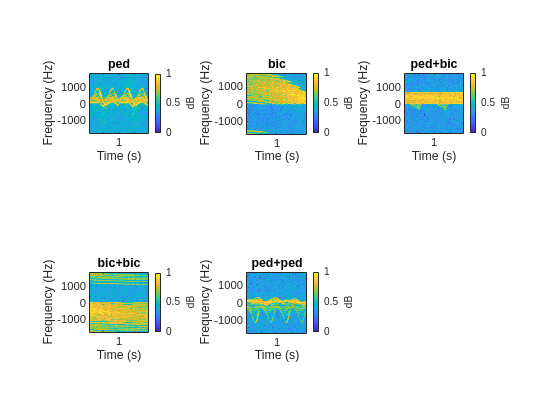Train the CNN that you created. You can view the accuracy and loss during the training process. In 30 epochs, the training process achieves almost 95% accuracy.

`trainedNetNoCar = trainNetwork(trainDataNoCar,trainLabelNoCar,layers,options);`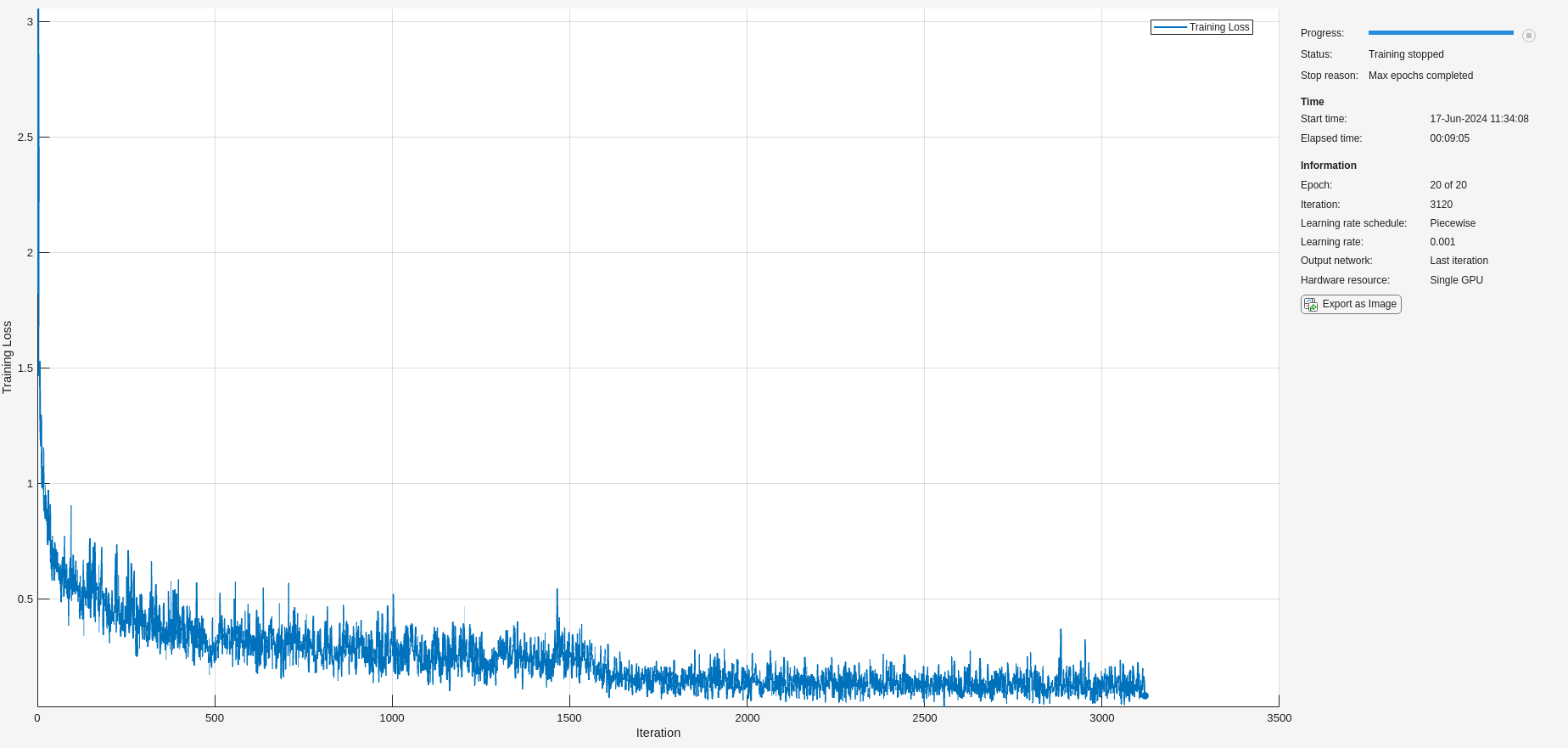Use the trained network and the `classify` function to obtain the predicted labels for the test data set `testDataNoCar`. The variable `predTestLabel` contains the network predictions. The network achieves about 95% accuracy for the test data set without the car noise.

```predTestLabel = classify(trainedNetNoCar,testDataNoCar); testAccuracy = mean(predTestLabel == testLabelNoCar)```
```testAccuracy = 0.9530 ```

Use a confusion matrix to view detailed information about prediction performance for each category. The confusion matrix for the trained network shows that, in each category, the network predicts the labels of the signals in the test data set with a high degree of accuracy.

```figure confusionchart(testLabelNoCar,predTestLabel);```### Classify Signatures with Car Noise

To analyze the effects of car noise, classify data containing car noise with the `trainedNetNoCar` network, which was trained without car noise.

Load the car-noise-corrupted test data set `testDataCarNoise.mat`.

`load(fullfile(tempdir,'PedBicCarData','testDataCarNoise.mat'))`

Input the car-noise-corrupted test data set to the network. The prediction accuracy for the test data set with the car noise drops significantly, to around 70%, because the network never saw training samples containing car noise.

```predTestLabel = classify(trainedNetNoCar,testDataCarNoise); testAccuracy = mean(predTestLabel == testLabelCarNoise)```
```testAccuracy = 0.7176 ```

The confusion matrix shows that most prediction errors occur when the network takes in scenes from the "pedestrian," "pedestrian+pedestrian," or "pedestrian+bicyclist" classes and classifies them as "bicyclist."

`confusionchart(testLabelCarNoise,predTestLabel);`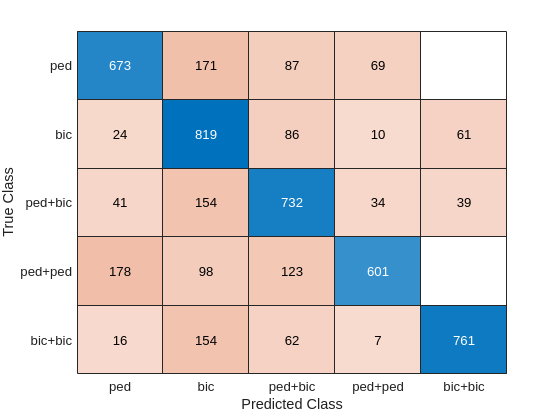Car noise significantly impedes the performance of the classifier. To solve this problem, train the CNN using data that contains car noise.

### Retrain CNN by Adding Car Noise to Training Data Set

Load the car-noise-corrupted training data set `trainDataCarNoise.mat`.

`load(fullfile(tempdir,'PedBicCarData','trainDataCarNoise.mat'))`

Retrain the network by using the car-noise-corrupted training data set. In 30 epochs, the training process achieves almost 90% accuracy.

`trainedNetCarNoise = trainNetwork(trainDataCarNoise,trainLabelCarNoise,layers,options);`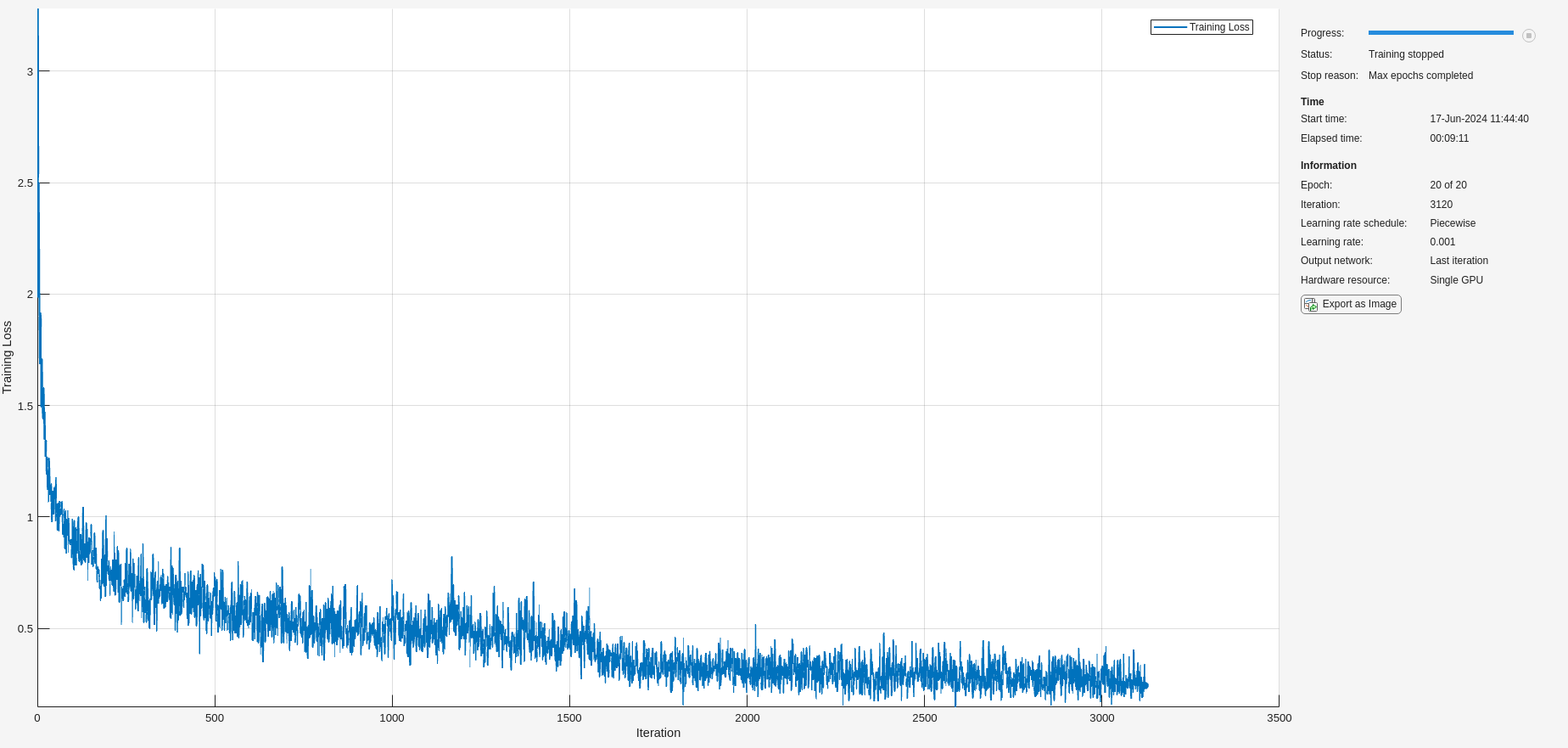Input the car-noise-corrupted test data set to the network `trainedNetCarNoise`. The prediction accuracy is about 87%, which is approximately 15% higher than the performance of the network trained without car noise samples.

```predTestLabel = classify(trainedNetCarNoise,testDataCarNoise); testAccuracy = mean(predTestLabel == testLabelCarNoise)```
```testAccuracy = 0.8728 ```

The confusion matrix shows that the network `trainedNetCarNoise` performs much better at predicting scenes with one pedestrian and scenes with two pedestrians.

`confusionchart(testLabelCarNoise,predTestLabel);`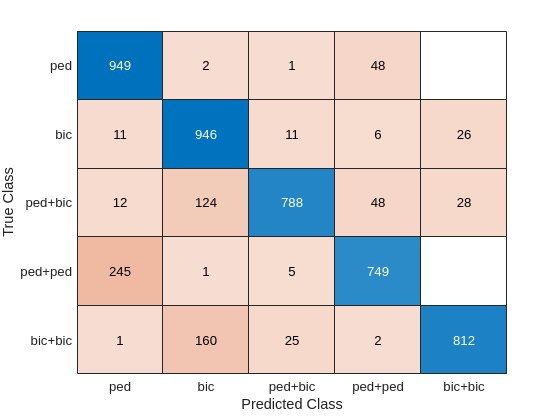### Case Study

To better understand the performance of the network, examine its performance in classifying overlapping signatures. This section is just for illustration. Due to the non-deterministic behavior of GPU training, you may not get the same classification results in this section when you rerun this example.

For example, signature #4 of the car-noise-corrupted test data, which does not have car noise, has two bicyclists with overlapping micro-Doppler signatures. The network correctly predicts that the scene has two bicyclists.

```k = 4; imagesc(T,F,testDataCarNoise(:,:,:,k)) axis xy xlabel('Time (s)') ylabel('Frequency (Hz)') title('Ground Truth: '+string(testLabelCarNoise(k))+', Prediction: '+string(predTestLabel(k)))```From the plot, the signature appears to be from only one bicyclist. Load the data `CaseStudyData.mat` of the two objects in the scene. The data contains return signals summed along the fast time. Apply the STFT to each signal.

```load CaseStudyData.mat M = 200; % FFT window length beta = 6; % window parameter w = kaiser(M,beta); % kaiser window R = floor(1.7*(M-1)/(beta+1)); % ROUGH estimate noverlap = M-R; % overlap length [Sc,F,T] = stft(x,1/Tsamp,'Window',w,'FFTLength',M*2,'OverlapLength',noverlap); for ii = 1:2 subplot(1,2,ii) imagesc(T,F,10*log10(abs(Sc(:,:,ii)))) xlabel('Time (s)') ylabel('Frequency (Hz)') title('Bicyclist') axis square xy title(['Bicyclist ' num2str(ii)]) c = colorbar; c.Label.String = 'dB'; end```The amplitudes of the Bicyclist 2 signature are much weaker than those of Bicyclist 1, and the signatures of the two bicyclists overlap. When they overlap, the two signatures cannot be visually distinguished. However, the neural network classifies the scene correctly.

Another case of interest is when the network confuses car noise with a bicyclist, as in signature #267 of the car-noise-corrupted test data:

```figure k = 267; imagesc(T,F,testDataCarNoise(:,:,:,k)) axis xy xlabel('Time (s)') ylabel('Frequency (Hz)') title('Ground Truth: '+string(testLabelCarNoise(k))+', Prediction: '+string(predTestLabel(k)))```The signature of the bicyclist is weak compared to that of the car, and the signature has spikes from the car noise. Because the signature of the car closely resembles that of a bicyclist pedaling or a pedestrian walking at a low speed, and has little micro-Doppler effect, there is a high possibility that the network will classify the scene incorrectly.

### References

 Chen, V. C. The Micro-Doppler Effect in Radar. London: Artech House, 2011.

 Gurbuz, S. Z., and Amin, M. G. "Radar-Based Human-Motion Recognition with Deep Learning: Promising Applications for Indoor Monitoring." IEEE Signal Processing Magazine. Vol. 36, Issue 4, 2019, pp. 16–28.

 Belgiovane, D., and C. C. Chen. "Micro-Doppler Characteristics of Pedestrians and Bicycles for Automotive Radar Sensors at 77 GHz." In 11th European Conference on Antennas and Propagation (EuCAP), 2912–2916. Paris: European Association on Antennas and Propagation, 2017.

 Angelov, A., A. Robertson, R. Murray-Smith, and F. Fioranelli. "Practical Classification of Different Moving Targets Using Automotive Radar and Deep Neural Networks." IET Radar, Sonar & Navigation. Vol. 12, Number 10, 2017, pp. 1082–1089.

 Parashar, K. N., M. C. Oveneke, M. Rykunov, H. Sahli, and A. Bourdoux. "Micro-Doppler Feature Extraction Using Convolutional Auto-Encoders for Low Latency Target Classification." In 2017 IEEE Radar Conference (RadarConf), 1739–1744. Seattle: IEEE, 2017.